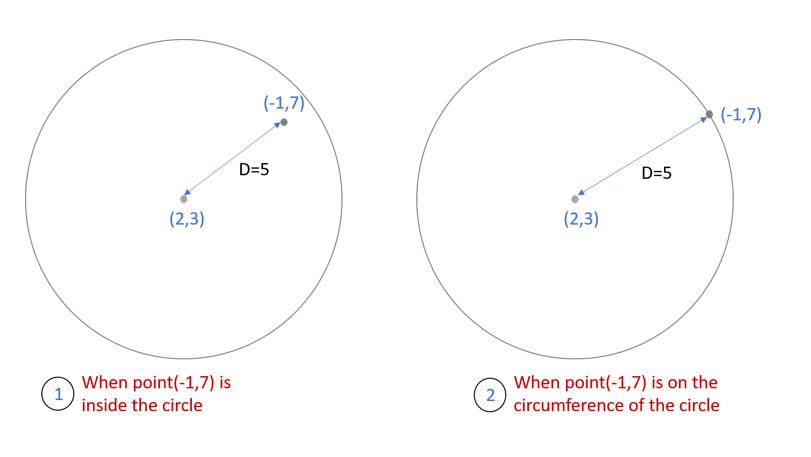GMAT Question of the Day - Daily to your Mailbox; hard ones only

 It is currently 15 Oct 2019, 21:39GMAT Club Daily Prep

Thank you for using the timer - this advanced tool can estimate your performance and suggest more practice questions. We have subscribed you to Daily Prep Questions via email.

Customized
for You

we will pick new questions that match your level based on your Timer History

Track

every week, we’ll send you an estimated GMAT score based on your performance

Practice
Pays

we will pick new questions that match your level based on your Timer History

Not interested in getting valuable practice questions and articles delivered to your email? No problem, unsubscribe here.In the coordinate system, the center of a circle lies at (2, 3)

 new topic post reply Question banks Downloads My Bookmarks Reviews Important topics
Author Message
Current StudentV
Joined: 28 May 2014
Posts: 515
GMAT 1: 730 Q49 V41In the coordinate system, the center of a circle lies at (2, 3)  [#permalink]

Show Tags

200:00

Difficulty:95% (hard)

Question Stats:38% (01:38) correct63% (01:36) wrongbased on 72 sessions

HideShow timer Statistics

In the coordinate system, the center of a circle lies at (2, 3). If point A with coordinates (-1, 7) does not lie outside the circle, which of the following points must lie inside the circle?

I. (0, 7)
II. (5, -1)
III. (-2, 7)

A) I only
B) II only
C) III only
D) I and II only
E) None of the above

_________________
DirectorP
Joined: 31 Jul 2017
Posts: 512
Location: Malaysia
Schools: INSEAD Jan '19
GMAT 1: 700 Q50 V33GPA: 3.95
WE: Consulting (Energy and Utilities)
Re: In the coordinate system, the center of a circle lies at (2, 3)  [#permalink]

Show Tags

saswata4s wrote:
In the coordinate system, the center of a circle lies at (2, 3). If point A with coordinates (-1, 7) does not lie outside the circle, which of the following points must lie inside the circle?

I. (0, 7)
II. (5, -1)
III. (-2, 7)

A) I only
B) II only
C) III only
D) I and II only
E) None of the above

The maximum distance which Point A can lie from centre = 5 (Its the distance from centre of circle to Point A).
(5,-1) & (-2,7) lies at the circle as their distance from Centre of Circle is 5. Distance from (0,7) to (2,3) is less than 5. Hence, (0,7) lies inside the circle.

Option A.

_________________
If my Post helps you in Gaining Knowledge, Help me with KUDOS.. !!
ManagerB
Joined: 04 Feb 2016
Posts: 61
Location: India
Concentration: Technology, Marketing
GMAT 1: 650 Q48 V32GPA: 4
WE: Sales (Computer Software)
Re: In the coordinate system, the center of a circle lies at (2, 3)  [#permalink]

Show Tags

r <=5 since the point must be on or inside the circle.
Option I gives R < 5. If R = 5 we do not know for certain if the point is inside the circle, hence option II is out. Option III is anyways greater than 5.

A.
_________________
GMAT1 650 Q48 V32.
InternB
Joined: 17 Jan 2018
Posts: 44
Schools: ISB '20 (A)
Re: In the coordinate system, the center of a circle lies at (2, 3)  [#permalink]

Show Tags

Origin, O - (2,3)
A (-1,7) - Does not lie outside the circle, implies it is either on the circle or inside the circle.

Distance between (2,3) and (-1,7) = 5
So, radius of the circle <= 5

Q) Must lie inside the circle, implies NEITHER outside the circle NOR on the circle.

D < 5 ===> D!>=5

Point 1 - Distance between (2,3) and (0,7) ===> Less than 5
Point 2 - Distance between (2,3) and (5,-1) ===> Equals to 5
Point 3 - Distance between (2,3) and (-2,7) ===> More than 5

Answer is Only I.

Option A.

saswata4s wrote:
In the coordinate system, the center of a circle lies at (2, 3). If point A with coordinates (-1, 7) does not lie outside the circle, which of the following points must lie inside the circle?

I. (0, 7)
II. (5, -1)
III. (-2, 7)

A) I only
B) II only
C) III only
D) I and II only
E) None of the above
e-GMAT RepresentativeV
Joined: 04 Jan 2015
Posts: 3074
Re: In the coordinate system, the center of a circle lies at (2, 3)  [#permalink]

Show Tags

1
1

Solution

If the point $$A (-1,7$$) does not lie outside the circle, then it can lie either on the circle or inside the circle as shown in the diagram.Let us denote the distance between the point $$A (-1,7)$$ and centre of the circle $$(2,3)$$ by $$D$$.
D= $$\sqrt{(2-(-1))^2+(3-7)^2}$$
D= 5

Now, when the distance of point A is 5, then we are uncertain whether it lies on the circle or inside the circle.

However, if the distance of any point from centre is less than 5 then we can infer, that the point is certainly inside the circle.

Let us find the distance of all the points given in options.

1- (0,7)

Distance= $$\sqrt{(2-0)^2+(3-7)^2}$$
Distance= $$\sqrt{20}$$, which is less than $$5$$.

Hence (0,7) lies inside the circle.

2- (5, -1)

Distance= $$\sqrt{(2-5)^2+(3-(-1))^2}$$
Distance= $$5$$

3- (-2, 7)

Distance= $$\sqrt{(2-(-2))^2+(3-7)^2}$$
Distance= $$\sqrt{32}$$, which is greater than $$5$$.

_________________Re: In the coordinate system, the center of a circle lies at (2, 3)   [#permalink] 25 Mar 2018, 11:51
Display posts from previous: Sort by

In the coordinate system, the center of a circle lies at (2, 3)

 new topic post reply Question banks Downloads My Bookmarks Reviews Important topics

 Powered by phpBB © phpBB Group | Emoji artwork provided by EmojiOne